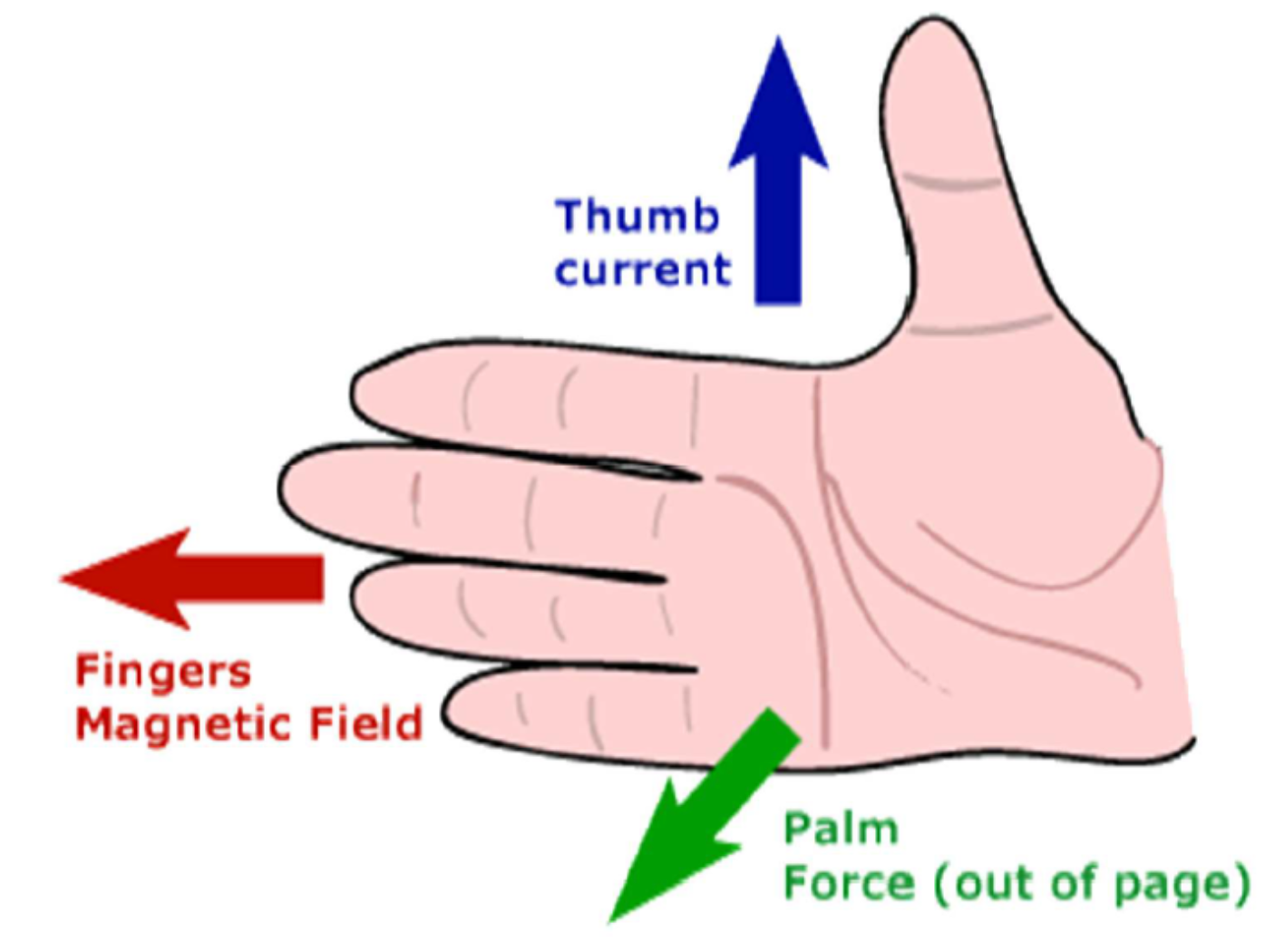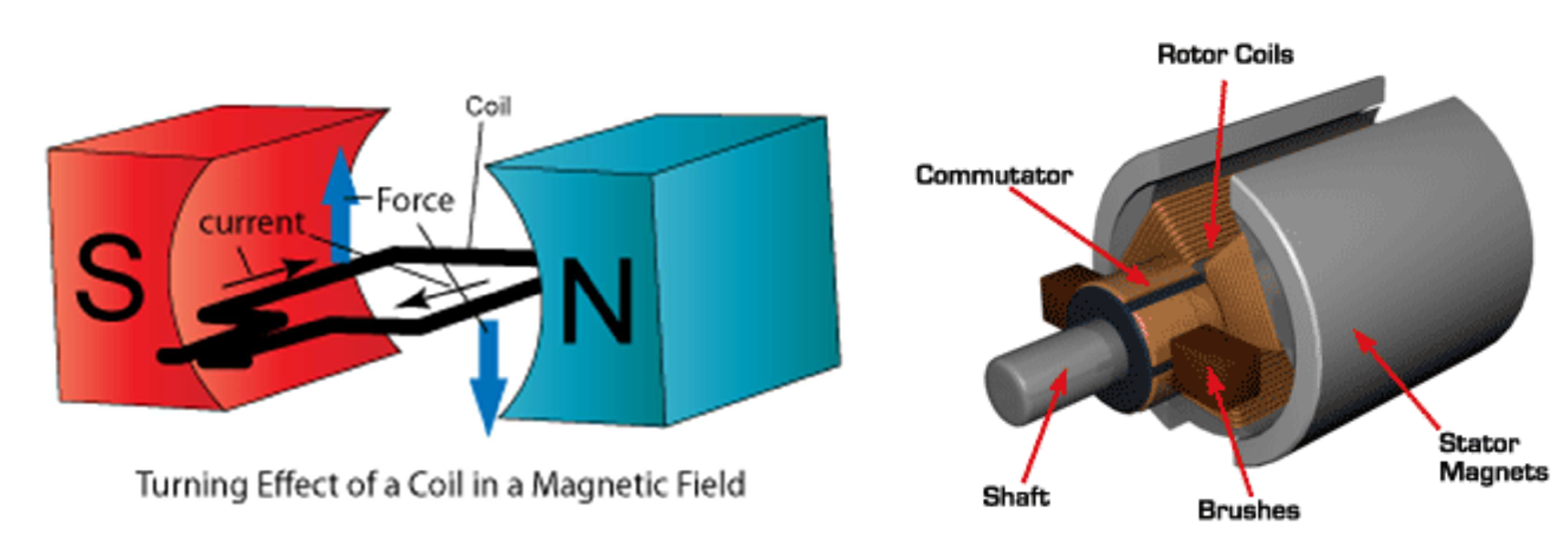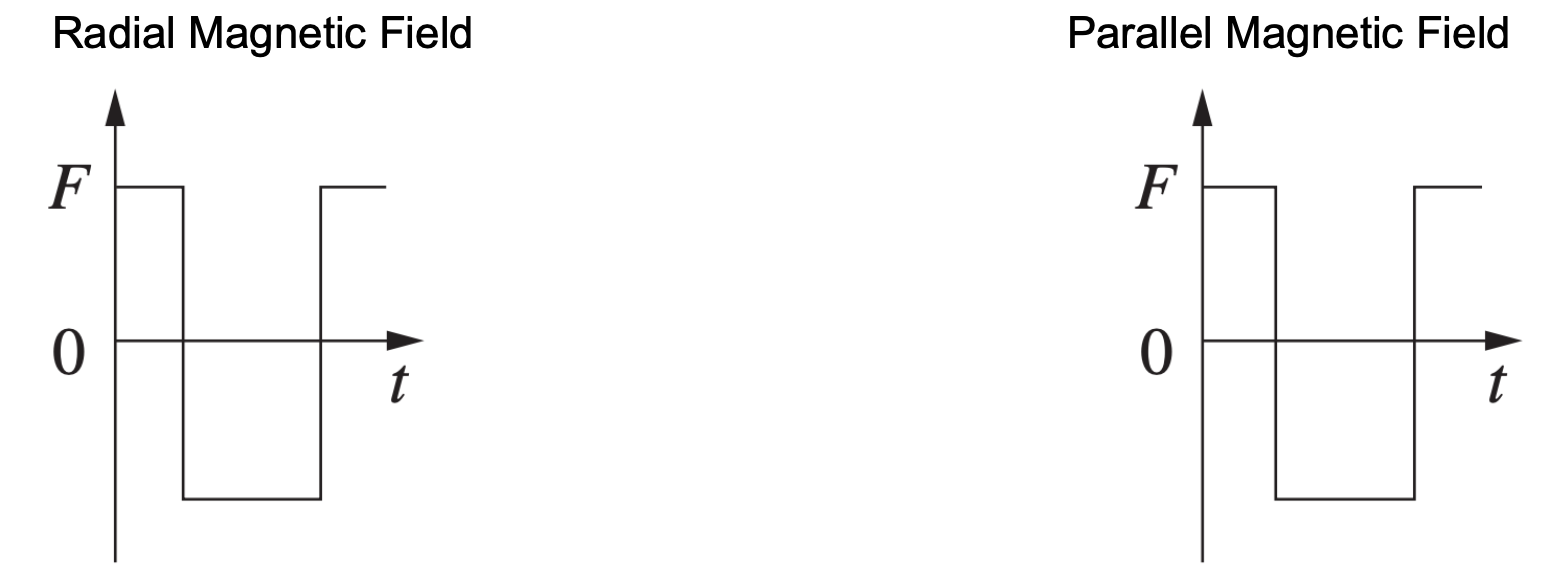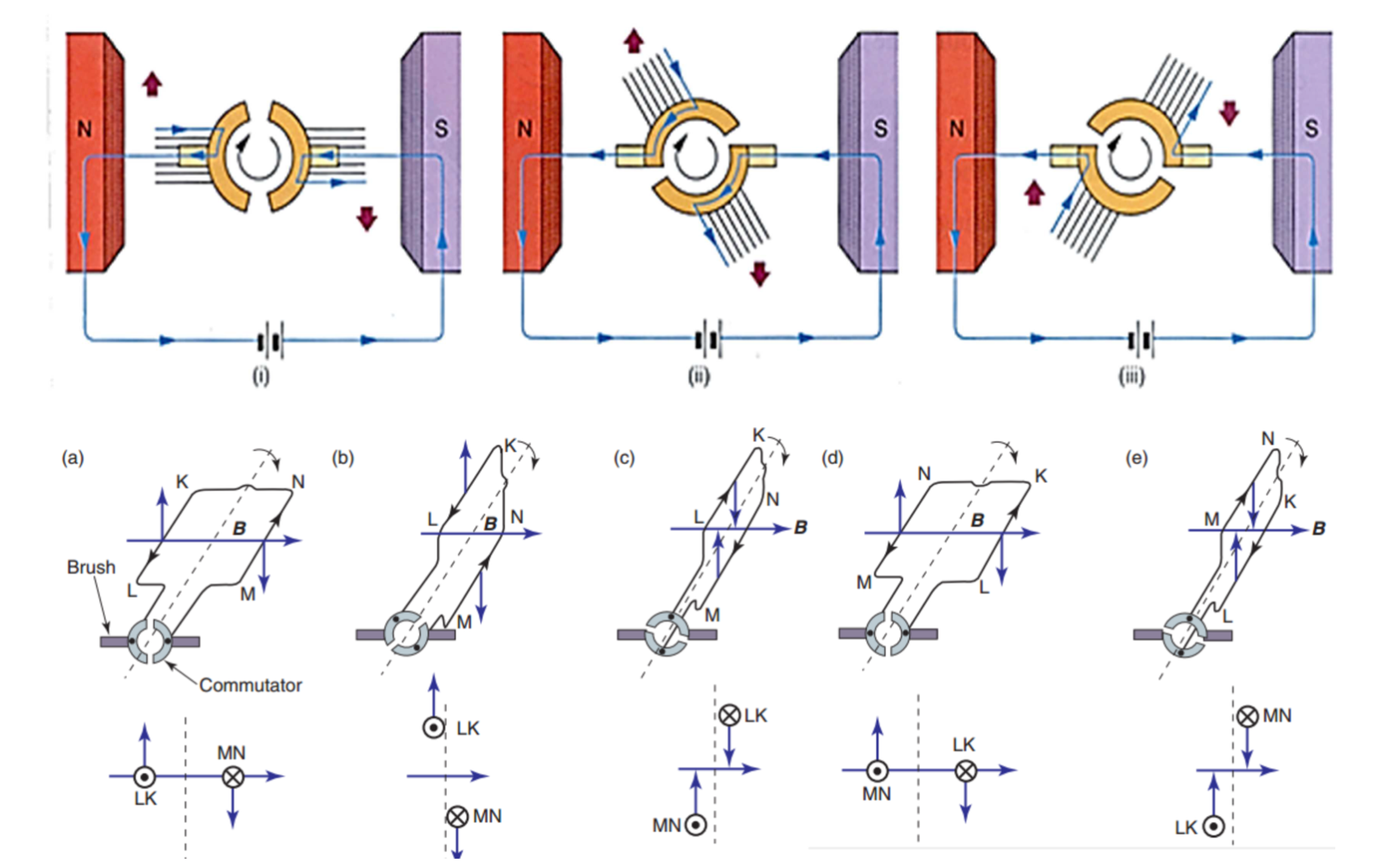# Operation of a Simple DC Motor

This is part of the HSC Physics syllabus under the topic Applications of the Motor Effect.

### HSC Physics Syllabus

• investigate the operation of a simple DC motor to analyse:

- the functions of its components

- production of a torque \tau = nIAB_(_|_) sin \theta

- effects of back emf (ACSPH108)

### How Does a Simple DC Motor Operate?

This video investigates the operation of a simple DC motor to analyse the functions of its components, the production of torque, and the effects of back emf.

### Operation of a Simple DC Motor

Recall that the motor effect states that a current-carrying conductor experiences a force in an external magnetic field.

$$F = BIl \sin \theta$$

We can use the right-hand palm rule to determine the direction of the motor force.The motor effect is the principle by which motors run. A motor is a device which converts electrical energy into mechanical (kinetic) energy. A DC motor runs on direct current (DC), that is, current that only flows in one direction.

When the DC battery is switched on, current flows through a coil, making it a current-carrying conductor. The coil then experiences a motor force due to an external magnetic field. This force develops a torque, causing the coil to rotate.### Components of a DC Motor

 Component Description Function Coil & Armature ·       Multiple turns (n) of wire are wound around the armature to form a coil. ·       The overall force resulted from the Motor Effect is proportional to the number of turns ·       Coil: carries current to produce mechanical energy as a result of the Motor Effect. ·       Armature refers to the entirety of the coil. It is effective the surface of the conductor which interacts with external magnetic flux to produce the Motor Effect and back emf. Axle ·       Located in the middle of the armature ·       The pivot point of applied torque and where the armature rotates about ·       Provides a point of rotation for the armature ·       Transfers the rotational mechanical energy to another appliance e.g. fan Magnets ·       Can either be permanent or electromagnetic. Electromagnetic is better as the strength of magnetic field can be changed by altering current ·       Usually radial magnets are used to produce a radial magnetic field. Radial magnetic fields ‘smooth’ out the rate of change in flux, such that the motor speed becomes more dependent on current size. ·       Produces an external magnetic field Split-ring commutator ·       Semi-crescent shaped, conductive material that is connected to the coil/armature ·       Rotates with the coil when the motor is running ·       Connects the armature with the brush and power source. ·       Reverses the current direction periodically (every half revolution) to keep torque direction constant and rotation continuous Brush ·       Conductive material e.g. carbon ·       Connected to external battery or other kinds of DC power source ·       Does not rotate with coil when the motor is running ·       Requires maintenance and replacement as frequent contact with split-ring commutators produce friction which wears out the material ·       Not physically fixed to split-ring commutators. Acts as a conductive connection between split-ring commutators & armature and power source (battery) Battery ·       Most common source of electromotive force (emf) which forces electrons to travel in one direction, hence producing direct current (DC) ·       Produces current à produces motor effect as the coil becomes a ‘current-carrying conductor’ in an external magnetic field

The diagram below illustrates the interaction of all these components. A radial magnet produces an external magnetic field, which exerts a motor force on the coil. Specifically, the left side of the coil experiences an upward force and the right side of the coil experiences a downward force. Consequently, the armature rotates clockwise as shown by the red arrow.

Notice the ‘split’ or gap is always perpendicular to the plane of the coil. Therefore, when the coil is in a vertical position, it loses contact with the DC supply as the ‘split’ becomes aligned with the brush. At this instance, the momentum of the coil carries it past the vertical position. Afterwards, the current direction with respect to the coil is reversed. This reverses the force direction, allowing for constant directional torque and thus rotation.The magnitude of motor force acting on the armature remains constant throughout its rotation within the magnetic field. This is true for both parallel and radial magnets as the angle between the coil and magnetic field lines is 90º in both cases.

The graphs below show how the magnitude of force is always constant, but the direction (sign) changes periodically (every 180°).### Torque in DC Motors

The total force acting on the armature equals to the force acting on a single turn of coils multiplied by the number of total turns.

$$F = nIlB \sin \theta$$

Recall that torque equals the product of the force and the distance from the pivot, with \theta being the angle between the lever arm and the force vector.

$$\tau = rF \sin \theta$$

Since in a DC motor, the motor force is always perpendicular to the current (by right hand palm rule), then it is also always perpendicular to the conductor. Hence, \theta = 90°, giving:

$$\tau = rF$$

In a DC motor, we consider r to be the distance from each arm to the midline of the coil. As such, r equals half the width (w)of the coil.

$$\tau = \frac{w}{2} F$$

Since the force is a motor force, then:

$$\tau = \frac{w}{2} nIlB \sin \theta$$

However, remember that there are two arms experiencing this torque, so the total torque will be:

$$\tau = 2 \times \frac{w}{2} nIlB \sin \theta$$

$$= wnIlB \sin \theta$$

$$= nIB \sin \theta \times (lw)$$

Since l (length of coil) times w (width of coil) equals the area (A) of the coil:

$$\tau = nIAB \sin \theta$$

where,

• n is the number of coil turns in the armature
• B is the magnetic field strength of flux density (in Tesla)
• I is the magnitude of current flowing through the armature (in Amperes)
• A is the total area created by the coil (in m^2)
• \theta is the angle between the area vector (a vector perpendicular to the plane of the coil) and the magnetic field lines.

### Why Do DC Motors Need a Split-Ring Commutator?

Direct current flowing through the coil leads to a constant direction force vector to act on the coil. This leads to the torque direction reversing every half turn, preventing a constant direction of rotation.

A split-ring commutator overcomes this problem. Every half turn, the pair of split-rings swap contact with the brushes. This reverses the current direction with respect to the coil. The overall current direction of the circuit remains the same. This allows the armature to continue rotating to complete its revolution.Diagram Explanation

(a) The armature is in a horizontal orientation with side LK experiencing an upward force and MN a downward force. In this position, the split-ring commutators are in contact with the brush, allowing the circuit to be complete.

(b) The armature is about to adopt a vertical orientation. Sides LK and MN experience the same force as in (a)

(c) The moment retained from before has allowed the armature to bypass the vertical position to adopt the new orientation in (c). Here, the split-ring commutator has reversed the current direction flowing through side LK and MN.

Notice the change of current direction in the second row of diagrams. This reversal of current keeps the torque constant which allows for continual motion. Additionally, notice the pair of split-ring commutators have switched their contact points with the brush.

(d) The armature has returned to its horizontal plane, but this time LK is experiencing a downward force, MN an upward force due to the reversal of current that has taken place before.

(e)The armature is about to reach a vertical orientation.

Previous section: ﻿Applications of Step-up and Step-down Transformers

﻿Next section: Parallel vs Radial Magnetic Fields